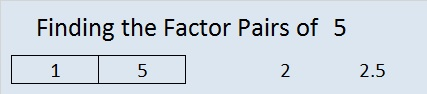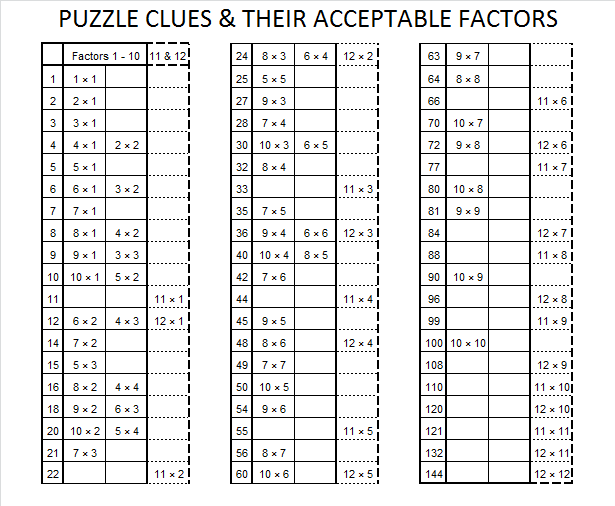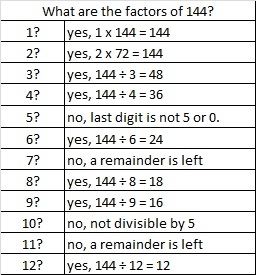# 5 Easy as 1-2-3

• 5 is a prime number.
• Prime factorization: 5 is prime.
• The exponent of prime number 5 is 1. Adding 1 to that exponent we get (1 + 1) = 2. Therefore 5 has exactly 2 factors.
• Factors of 5: 1, 5
• Factor pairs: 5 = 1 x 5
• 5 has no square factors that allow its square root to be simplified. √5 ≈ 2.236.How do we know that 5 is a prime number? If 5 were not a prime number, then it would be divisible by at least one prime number less than or equal to √5 ≈ 2.2. Since 5 cannot be divided evenly by 2, we know that 5 is a prime number.

5 is the only number that is the sum of ALL the prime numbers less than itself.

2² + 1² = 5 and 3² + 4² = 5² so 5 is the smallest Pythagorean triple hypotenuse.

When 5 is a clue in a FIND THE FACTORS puzzle, use 1 for one of the factors and 5 for the other.

Being able to identify factors of a whole number is a very important skill in mathematics.  It is a skill that is commonly used in many areas of mathematics ranging from reducing fractions to solving differential equations.  The Find the Factors puzzles can help make that skill second nature.Click 10 Factors 2013-11-11 for more puzzles.

To solve the puzzles, we are only interested in the limited set of factors that are represented in the following table:What about all the other factors of these numbers?  And what about all the other whole numbers not on the chart?  How do you find ALL of the factors of a given whole number?  For example, suppose you were asked to find all of the factors of 435.  Some people might notice right away that it is divisible by 5 because its last digit is 5.  While that is true, beginning with 5 is not the best place to start because there is an advantage in considering all possible factors in an organized way.  When you are asked to find ALL of the factors of any number, starting at 1 will make finding all of the factors as easy as 1-2-3. So what are the factors of 435?  Using a calculator, I notice that the square root of 435 is about 20.85.  That means I can find absolutely all of the factors of 435 by considering as  divisors just the whole numbers from 1 to 20!  Each factor will have a partner that is greater than 20 but will be found at the same time with these few short calculations. To demonstrate my thinking process, I will put each possible factor from 1 to 20 in a chart and write my thoughts as I consider each one.As you may notice, once a possible factor is eliminated, it is not necessary to do any actual division by ANY of the multiples of that number. (4, 6, 8, 10, 12, 14, 16, 18, and 20 are all multiples of 2, which was not a factor, so I didn’t actually divide 435 by any of those multiples.)

As I carefully consider each possible factor, I only WRITE DOWN a number if it is an actual factor.  Therefore, with only a little bit of effort I would list ALL of the factors of 435 in one tidy list: 1 x 435, 3 x 145, 5 x 87, 15 x 29.

See, it was as easy as 1-2-3!  Now let’s find all of the factors of 144.Even though 144 is less than 435, it has more factors. One of its factors is paired with itself because the square root of 144  is 12.  That fact is also the signal that we can stop looking for more factors, and we can list all the factors of 144 on the following chart:There are 8 multiplication facts that produce 144, but 12 x 12 = 144 is the only fact we consider when solving a Find the Factors 1-12 puzzle with 144 as one of the clues. In every other case one of the pair of numbers in the multiplication fact will be greater than 12 and not eligible to be written in the factor row or factor column. However in solving mathematical problems, any of the factors of a whole number could be the star of the show. Knowing how to find those factors is indeed an important skill and is as easy as 1-2-3.